Courses

# The Direct Stiffness Method: Truss Analysis - 2 GATE Notes | EduRev

## GATE : The Direct Stiffness Method: Truss Analysis - 2 GATE Notes | EduRev

The document The Direct Stiffness Method: Truss Analysis - 2 GATE Notes | EduRev is a part of the GATE Course Structural Analysis.
All you need of GATE at this link: GATE

Transformation from Local to Global Co-ordinate System.

Displacement Transformation Matrix

A truss member is shown in local and global co ordinate system in Fig. 24.6. Let x'y'z' be in local co ordinate system and xyz be the global co ordinate system.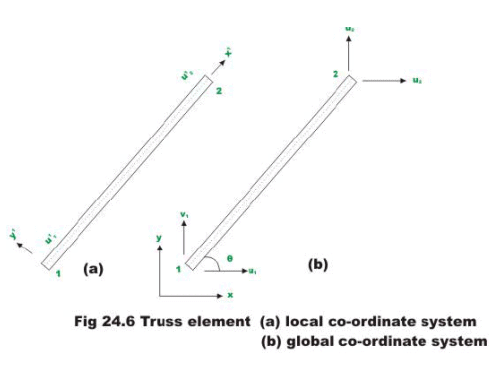The nodes of the truss member be identified by 1 and 2. Let u'1 and u'2 be the displacement of nodes 1 and 2 in local co ordinate system. In global co ordinate system, each node has two degrees of freedom. Thus, u1 , v2 and u2, v2 are the nodal displacements at nodes 1 and 2 respectively along x - and y- directions. Let the truss member be inclined to x axis by θ as shown in figure. It is observed from the figure that u'1 is equal to the projection of u1 on x' axis plus projection of v1 on x' -axis. Thus, (vide Fig. 24.7)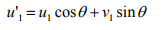(24.8a)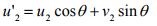(24.8b)

This may be written as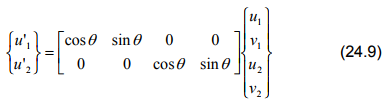Introducing direction cosines l = cos θ ; m = sin θ the above equation is written as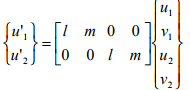(24.10a)

Or,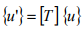(24.10b)

In the above equation [T] is the displacement transformation matrix which transforms the four global displacement components to two displacement component in local coordinate system.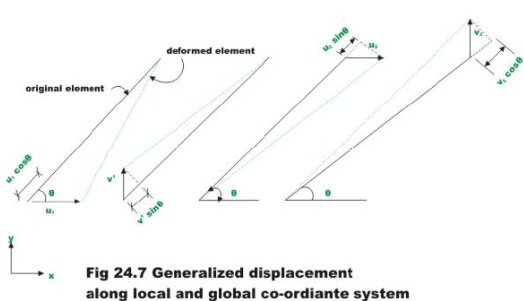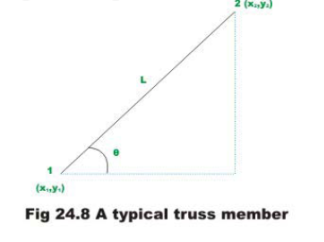Let co-ordinates of node 1 be (x1,y1) and node 2 be (x2,y2). Now from Fig. 24.8,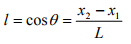(24.11a)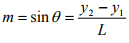(24.11b)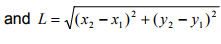(24.11c)

Force transformation matrix

Let P'1, P'2 be the forces in a truss member at node 1 and 2 respectively producing displacements u'1 and u'2 in the local co-ordinate system and P1, P2, P3, P4  be the force in global co-ordinate system at node 1 and 2  respectively producing displacements u1, v1 and u2, v2 (refer Fig. 24.9a-d).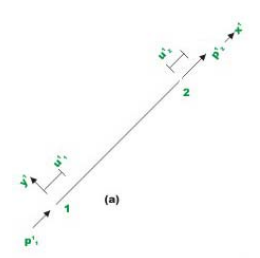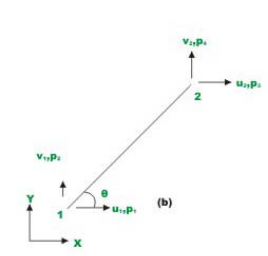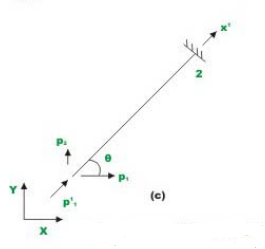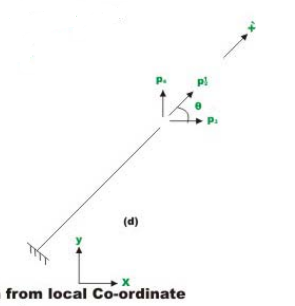► A
Fig 24.9 Forces transformation from local Co-ordinate system to global Co-ordinate system

Referring to fig. 24.9c, the relation between p'1 and p1, may be written as,

p1 = p'1 cosθ                                       (24.12a)

p2 = p'1 sinθ                                        (24.12b)

Similarly referring to Fig. 24.9d, yields

p3 = p'2 cosθ                                    (24.12c)

p4 = p'2 sinθ                                     (24.12d)

Now the relation between forces in the global and local co-ordinate system may be written as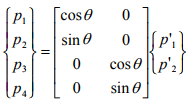(24.13)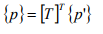(24.14)

where matrix {p} stands for global components of force and matrix {p'} are the components of forces in the local co-ordinate system. The superscript T stands for the transpose of the matrix. The equation (24.14) transforms the forces in the local co-ordinate system to the forces in global co-ordinate system. This is accomplished by force transformation matrix [T]T . Force transformation matrix is the transpose of displacement transformation matrix.

Member Global Stiffness Matrix

From equation (24.6b) we have,

{p'} = [k'] {u'}

Substituting for {p'}in equation (24.14), we get

{p} = [T]T [k'] {u'}                             (24.15)

Making use of the equation (24.10b), the above equation may be written as

{p} = [T]T [k'][T]{u}                                                 (24.16)

{p} = [k] {u}                                                            (24.17)

Equation (24.17) represents the member load displacement relation in global co- ordinates and thus [k] is the member global stiffness matrix. Thus,

{k} = [T]T [k'][T]                                                      (24.18)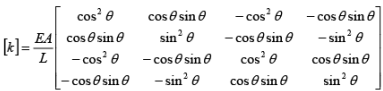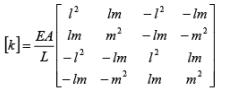(24.19)

Each component of the member stiffness matrix kij [k] in global co-ordinates represents the force in x -or y-directions at the end i required to cause a unit displacement along  x − or y − directions at end j .

We obtained the member stiffness matrix in the global co-ordinates by transforming the member stiffness matrix in the local co-ordinates. The member stiffness matrix in global co-ordinates can also be derived from basic principles in a direct method. Now give a unit displacement along x -direction at node 1 of the truss member. Due to this unit displacement (see Fig. 24.10) the member length gets changed in the axial direction by an amount equal to Δl1 = cosθ . This axial change in length is related to the force in the member in two axial directions by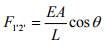(24.20a)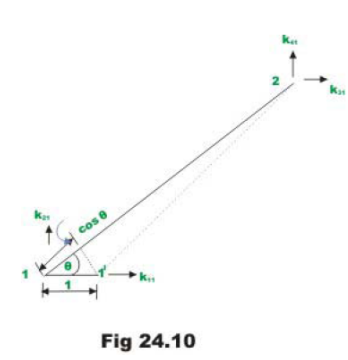This force may be resolved along u1 and u2 directions. Thus horizontal component of force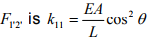(24.20b)

Vertical component of force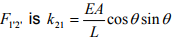(24.20c)

The forces at the node 2 are readily found from static equilibrium. Thus,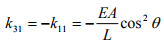(24.20d)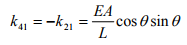(24.20e)

The above four stiffness coefficients constitute the first column of a stiffness matrix in the global co-ordinate system. Similarly, remaining columns of the stiffness matrix may be obtained.

Offer running on EduRev: Apply code STAYHOME200 to get INR 200 off on our premium plan EduRev Infinity!

## Structural Analysis

30 videos|122 docs|28 tests

,

,

,

,

,

,

,

,

,

,

,

,

,

,

,

,

,

,

,

,

,

;